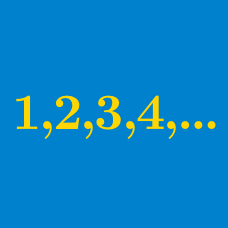Algebra

# Arithmetic Progressions: Level 3 Challenges

$\left (1 + 3 + 5 + \ldots + p^\text{th} \right ) \\ + \\ \left (1 + 3 + 5 + \ldots + q^\text{th} \right ) \\ = \\ \left (1 + 3 + 5 + \ldots + r^\text{th} \right )$

Given the above equation for positive integers $p,q,r$ with $p^\text{th}$ term greater than 39.

What is the smallest possible value of the expression below?

$\large p^\text{th} \ \text{term } + q^\text{th} \ \text{term } + r^\text{th} \ \text{term }$

$\dfrac{a(q-r)}{p} + \dfrac{b(r-p)}{q} + \dfrac{c(p-q)}{r}$

In an arithmetic progression, the sum of the first $p, q, r$ terms are $a, b, c$ respectively. Compute the expression above.

Consider an arithmetic progression with 2 and 101 as its first term and last term respectively. If the sum of the first 5 terms of this arithmetic progression is 40, find the sum of the last 5 terms of this progression.

$\begin{array}{llllll} A_1 : & 2, & 9, & 16, \ldots , & 2 + (1000-1) \times 7 \\ A_2: & 3, & 12 , & 21, \ldots, & 3 + (1000-1) \times 9? \\ \end{array}$

How many integers appear in both of the following arithmetic progressions above?

Details and assumptions

Since 2 appears in $A_1$ but not in $A_2$, it does not appear in both of the arithmetic progressions.

$\large \frac ab \ , \ ab \ , \ a -b \ , \ a+b$

Above shows real numbers that belong to an arithmetic progression in order. Find the next term of this sequence.

×